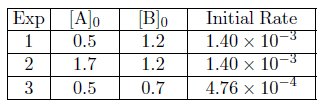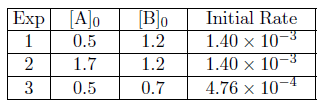# Problem: What is the rate law for the reaction A + B → C? The following data were collected.1. rate = 9.72 x 10-4 [A]0 [B]22. rate = 5.6 x 10-3 [A]2 [B]03. rate = 1.94 x 10-3 [A]0 [B]24. rate = 4.67 x 10-3 [A]2 [B]15. rate = 2.33 x 10-3 [A]0 [B]2

###### FREE Expert Solution

We’re being asked to determine the rate law for the reaction using the following data:

A + B → CRecall that the rate law only focuses on the reactant concentrations and has a general form of:

k = rate constant
A & B = reactants
x & y = reactant orders

We’re going to calculate the rate law and the rate constant using the following steps:

95% (296 ratings)###### Problem Details

What is the rate law for the reaction A + B → C? The following data were collected.

1. rate = 9.72 x 10-4 [A]0 [B]2

2. rate = 5.6 x 10-3 [A]2 [B]0

3. rate = 1.94 x 10-3 [A]0 [B]2

4. rate = 4.67 x 10-3 [A]2 [B]1

5. rate = 2.33 x 10-3 [A]0 [B]2